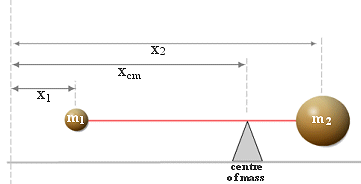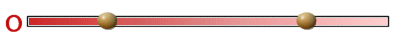Go to contentThe center of mass of a system is a specific point at which, for many purposes, the system's mass behaves as if it were concentrated. The center of mass is a function only of the positions and masses of the particles that comprise the system.
In the diagram opposite
\qquad (m_1+m_2) \times x_{cm} = (m_1 \times x_1 ) + (m_2 \times x_2 )
In the case of a rigid body, the position of its center of mass is fixed in relation to the object (but not necessarily in contact with it). In the context of an entirely uniform gravitational field, the center of mass is often called the center of gravity — the point where gravity can be said to act.
The center of mass of a body does not always coincide with its intuitive geometric center, and one can exploit this freedom. Engineers try to design a sport car center of gravity as low as possible to make the car handle better. When high jumpers perform a "Fosbury Flop", they bend their body in such a way that it is possible for the jumper to clear the bar while his or her center of mass does not.You can get a better display of the maths by downloading special TeX fonts from jsMath. In the meantime, we will do the best we can with the fonts you have, but it may not be pretty and some equations may not be rendered correctly.

## Glossary

### body

an object with both mass and size that cannot be taken to be a particle

### function

A rule that connects one value in one set with one and only one value in another set.

### geometric

A sequence where each term is obtained by multiplying the previous one by a constant.

### gravity

the force that the Earth exerts on all objects. It acts towards the centre of the Earth.
If the mass of the object is m kg then this force is defined to be mg Newtons, where g is the acceleration due to gravity.

### mass

a measure of the quantity of matter in an object

### rigid body

A model for an object with a shape that cannot be deformed, so that the distance between any two points within it remain constant, no matter what forces are applied.

### union

The union of two sets A and B is the set containing all the elements of A and B.

Full Glossary List

## This question appears in the following syllabi:

SyllabusModuleSectionTopicExam Year
AQA A-Level (UK - Pre-2017)M2Centres of MassSystems of particles-
AQA A2 Further Maths 2017MechanicsCentre of Mass and MomentsComposite Plane Figures-
AQA AS/A2 Further Maths 2017MechanicsCentre of Mass and MomentsComposite Plane Figures-
CCEA A-Level (NI)M3Centres of MassSystems of particles-
CIE A-Level (UK)M2Centres of MassSystems of particles-
Edexcel A-Level (UK - Pre-2017)M2Centres of MassSystems of particles-
Edexcel AS Further Maths 2017Further Mechanics 2Centre of Mass of Plane FiguresComposite Plane Figures-
Edexcel AS/A2 Further Maths 2017Further Mechanics 2Centre of Mass of Plane FiguresComposite Plane Figures-
OCR A-Level (UK - Pre-2017)M2Centres of MassSystems of particles-
OCR A2 Further Maths 2017MechanicsCentre of MassComposite Plane Figures-
OCR MEI AS Further Maths 2017Mechanics ACentre of MassComposite Plane Figures-
OCR-MEI A-Level (UK - Pre-2017)M2Centres of MassSystems of particles-
Universal (all site questions)CCentres of MassSystems of particles-
WJEC A-Level (Wales)M1Centres of MassSystems of particles-